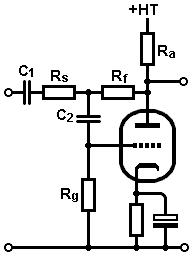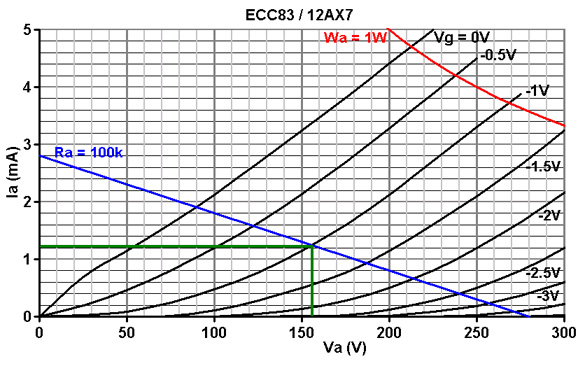# The Valve Wizard

How to design valve guitar amplifiers!

Triodes with Local Negative FeedbackApplying local feedback in guitar amplifiers is fairly uncommon since most strive to maximise the available gain. It is practically de rigueur for hi-fi as it reduces distortion and output impedance, widens and flatten bandwidth, and high levels of gain are rarely needed. A stage with local feedback also makes quite a good mixer, which is useful for both guitar and hi-fi.

The higher the opan-loop gain (that is, the higher the gain before feedback is added) the greater the loop gain and therefore the greater the distortion reduction and other benefits. Higher loop gain also makes the simplified formulae more accurate. High mu valves such as the ECC81 (12AT7) and ECC83 (12AX7) are therefore often used.

Design begins in the same way as for a typical triode gain stage. Choose a suitable HT and anode load (Ra), usually in the region of 100k, or whatever value gives fairly high gain without being so large that it will be too easily loaded by the feedback components. Draw a load line:Next choose a bias point in the usual way. In this case -1V looks good, for a quiescent anode current of 1.2mA. Use Ohm's law to find the value of bias resistor (Rk):
1 / 0.0012 = 833 ohms.
The nearest standard is 820R.

Although the cathode could be left un-bypassed it would reduce the open-loop gain and increase the output impedance, so we should add a cathode bypass capacitor.

The capacitor needs to have a low reactance at all audio frequencies or phase shift and reduced open-loop gain will impare stability and introduce weird resonance effects. For a low roll-off of 10Hz:
C = 1 / (2 * pi * f * Rk)
C = 1 / (2 * pi * 10 * 820)
= 19uF
The nearest standard is 22uF, although an arbitrarily large value could be used. LED biasing would of course avoid all problems of phase shift.

Open-loop gain and output impedance: We can now calculate the open-loop gain of the stage, either from the load line or by using the formula:
Ao = -(Ra * mu)/(Ra + ra)
Ao = -(100000 * 100)/(100000 + 55000)
= -64.5
Normally we would ignore the minus sign but in this case it is worth nothing that the stage is inverting and therefore the feedback must be negative. Positive feedback would result in oscillation.

Also find the output impedance from the anode before feedback is applied, using:
Zoa = Ra || ra
Zoa = 100000 || 55000
= 35.5K

Applying feedback: We can now find values for the grid resistor (Rg) and feeback resistor (Rf) if we know what closed-loop gain we want to end up with. If we would like a closed-loop gain of 10, we could either choose Rg or Rf first.
Since the feedback resistor is effectively in parallel with the anode resistor, we should make its value large or it will load down the valve. Rf should therefore be at least three times larger than Ra, and preferably more. If we choose 1Meg we can be sure the open-loop gain will be hardly affected.
If we assume the stage is an ideal amplifier with zero output impedance and infinite open-loop gain we can find an approximate value for Rg:
Av = Rf / Rg
so; Rg = Rf / A
Rg = 1000000 / 10
= 100k.

We can now use more accurate formulae to find out what the true performance of the circuit will be:
Zout = (Rg + Rf) * ra / (Rg + Rf + ra (mu+1))
Zout = (100000 + 1000000) * 55000 / (100000 + 1000000 + 55000 * (101))
= 9k
This is very low for a valve stage, but still about ten times greater than that achievable with a cathode follower.

The input impedance will be:
Zin = ra(Rg + Rf) + Ra(ra + Rg + Rf + muRg) / (ra + Ra + muRa)
Zin = 55000(100000 + 1000000) + 100000(55000 + 100000 + 1000000 + 100*100000) / (55000 + 100000 + 100*100000)
=116k

This is usually approximated as Zin = Rg. This low input impedance is one of the major drawbacks of this circuit, since it would need to be driven from a fairly low impedance source. We could increase Rg and Rf greatly to improve things, but that would increase resistor noise. Therefore the circuit is most useful when set to very low levels of closed-loop gain (e.g., less than 5) so that Rg can approach 1Meg.

The true gain of the stage will be:
Av = (Zoa + Ao * Rf) / (Rg + Rf + Zoa - Rg * Ao)
Av = (35500 + -64.5 * 1000000) / (100000 + 1000000 + 35500 - 100000 * -64.5)
= -8.5
In most cases this gain would be close enough to our aim of 10, otherwise some tweaking of values will be needed.

Coupling capacitors: The output coupling capacitor should not be used to control the output frequency response as it normally could, because this can cause weird resonance effects or ringing, because it is within the feedback loop.
It should be chosen using the value of feedback resistor, and should have a low reactance at all audio frequencies. For a low roll-off of 1Hz:
C = 1 / (2 * pi * f * Rf)
C = 1 / (2 * pi * 1 * Rf)
= 159nF
So 150nF would do, and we could get away with 100nF also, for a roll-off of 1.6Hz, or 47nF for a roll-off of 3.4Hz.The low-roll of the stage can be set with the input coupling capacitor, which is chosen in conjunction with the input impedance.